Silo

Outer perimeter of silo is 32 m. Concrete wall is 35 cm thick. What is diameter and area of inside floor of silo?

Result

d =  9.49 m
S =  70.67 m2

Solution:Leave us a comment of example and its solution (i.e. if it is still somewhat unclear...):Be the first to comment!Next similar examples:

1. Circular lawnAround a circular lawn area is 2 m wide sidewalk. The outer edge of the sidewalk is curb whose width is 2 m. Curbstone and the inner side of the sidewalk together form a concentric circles. Calculate the area of the circular lawn and the result round to 1
2. CircleWhat is the radius of the circle whose perimeter is 6 cm?
3. Circle r,DCalculate the diameter and radius of the circle if it has length 52.45 cm.
4. FlowerbedIn the park there is a large circular flowerbed with a diameter of 12 m. Jakub circulated him ten times and the smaller Vojtoseven times. How many meters each went by and how many meters did Jakub run more than Vojta?
5. MineWheel in traction tower has a diameter 5 m. How many meters will perform an elevator cabin if wheel rotates in the same direction 49 times?
6. Wheels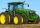Small tractor wheel with a diameter of 60 cm must be rotated 8 times to overcome some pathway. How many times must turn the big tractor wheel with a radius 60 cm to overcome the same distance?
7. Circle - simpleCalculate the area of a circle in dm2, if its circumference is 31.4 cm.Determine the radius of the circle, if its perimeter and area is the same number.
9. Circle inscribed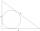Calculate the perimeter and area of a circle inscribed in a triangle measuring 3 , 4 and 5 cm.
10. Folded square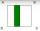ABCD is a square. The square is folded on the midpoint of AB and A is folded onto the fold, creating a shaded region. The perimiter of the shaded figure is 75. Find the area of square ABCD
11. Four circles1) Calculate the circle radius if its area is 400 cm square 2) Calculate the radius of the circle whose circumference is 400 cm. 3) Calculate circle circumference if its area is 400 cm square 4) Calculate the circle's area if perimeter 400 cm.
12. Area to perimeterCalculate circle circumference if its area is 254.34cm2
13. Theorem proveWe want to prove the sentence: If the natural number n is divisible by six, then n is divisible by three. From what assumption we started?
14. CableCable consists of 8 strands, each strand consists of 12 wires with diameter d = 0.5 mm. Calculate the cross-section of the cable.
15. AceThe length of segment AB is 24 cm and the point M and N divided it into thirds. Calculate the circumference and area of this shape.
16. Squaring the Circle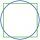Calculating side of the square with the same area as the circle of radius 18.
17. Annulus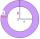Two concentric circles form an annulus of width 10 cm. The radius of the smaller circle is 20 cm. Calculate the content area of annulus.